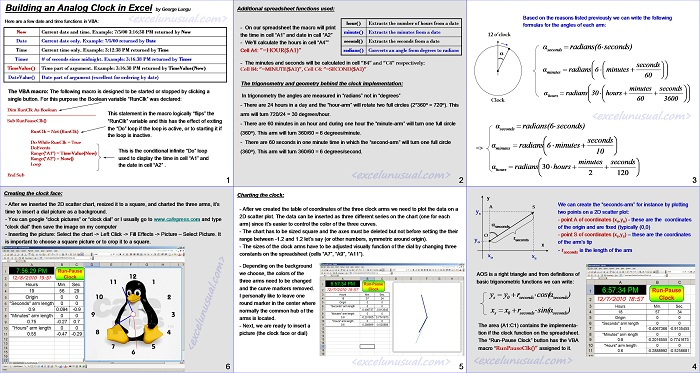# How to Make an Analog Clock in Excel

Hello folks, here is a tutorial about building a clock in Excel.

Enjoy![sociallocker][/sociallocker]

## Building a live Excel clock

by George Lungu

Here are a few date and time functions in VBA:

Now  : Current date and time.   Example: 7/5/00 3:16:38 PM returned by Now
Date  : Current date only.           Example: 7/5/00 returned by Date
Time : Current time only.           Example: 3:12:38 PM returned by Time
Timer # of seconds since midnight. Example: 3:16:38 PM returned by Timer
TimeValue() Time part of argument. Example: 3:16:38 PM returned by TimeValue(Now)
DateValue() Date part of argument (excellent for ordering by date)

### The VBA macro:

The following macro is designed to be started or stopped by clicking a single button. For this purpose the Boolean variable “RunClk” was declared:

Dim RunClk As Boolean

———————————————————–
Sub RunPauseClk() “RunClk”
RunClk = Not (RunClk)                 ‘This statement in the macro logically “flips” the variable and this has the effect of exiting the “Do” loop if the loop is active, or to starting it if the loop is inactive.
Do While RunClk = True             ‘This is the conditional infinite “Do” loop used to display the time in cell “A1” and the date in cell “A2”
DoEvents
Range(“A1”) = TimeValue(Now)
Range(“A2”) = Now()
Loop                ‘end statement of “Do” loop
End Sub          ‘end statement of the macro

### Additional spreadsheet functions used:

hour() – Extracts the number of hours from a date.
minute() – Extracts the minutes from a date.
second() – Extracts the seconds from a date.
radians() – Converts an angle from degrees to radians.

– On our spreadsheet the macro will print the time in cell “A1” and date in call “A2”
– We’ll calculate the hours in cell “A4””

Cell A4: ”=HOUR(\$A1)”
– The minutes and seconds will be calculated in cell “B4” and “C4” respectively:
Cell B4: “=MINUTE(\$A1)”, Cell C4: “=SECOND(\$A1)”

### The trigonometry and geometry behind the clock implementation:

In trigonometry the angles are measured in “radians” not in “degrees”

– There are 24 hours in a day and the “hour-arm” will rotate two full circles (2*360o = 720o). This arm will turn 720/24 = 30 degrees/hour.

– There are 60 minutes in an hour and during one hour the “minute-arm” will turn one full circle (360o). This arm will turn 360/60 = 6 degrees/minute.

– There are 60 seconds in one minute time in which the “second-arm” will turn one full circle (360o). This arm will turn 360/60 = 6 degrees/second.

<excelunusual.com > 2

### Based on the reasons listed previously we can write the following formulas for the angles of each arm:

We can create the “seconds-arm” for instance by plotting two points on a 2D scatter plot:

– point A of coordinates (xo,yo) – these are the coordinates of the origin and are fixed (typically (0,0)

– point S of coordinates (xs,ys) – these are the coordinates of the arm’s tip

– rseconds is the length of the arm

xo xs

AOS is a right triangle and from definitions of basic trigonometric functions we can write:

Y s= Y 0+R seconds*cos(α )
X s= X 0+R seconds*sin(α )

The area (A1:C1) contains the implementation if the clock function on the spreadsheet.
The “Run-Pause Clock” button has the VBA macro “RunPauseClk()” assigned to it.

<excelunusual.com > 4

### Charting the clock:

– After we created the table of coordinates of the three clock arms we need to plot the data on a 2D scatter plot. The data can be inserted as three different series on the chart (one for each arm) since it’s easier to control the color of the three curves.

– The chart has to be sized square and the axes must be deleted but not before setting the their range between -1.2 and 1.2 let’s say (or other numbers, symmetric around origin).

– The sizes of the clock arms have to be adjusted visually function of the dial by changing three constants on the spreadsheet (cells “A7”, “A9”, “A11”).

– Depending on the background we choose, the colors of the three arms need to be changed and the curve markers removed.

-I personally like to leave one round marker in the center where normally the common hub of the arms is located.

– Next, we are ready to insert a picture (the clock face or dial)

<excelunusual.com > 5

### Creating the clock face:

– After we inserted the 2D scatter chart, resized it to a square, and charted the three arms, it’s time to insert a dial picture as a background.

– You can google “clock pictures” or “clock dial” or I usually go to www.cafepress.com and type “clock dial” then save the image on my computer

– Inserting the picture: Select the chart -> Left Click -> Fill Effects -> Picture – Select Picture. It is important to choose a square picture or to crop it to a square.

by George Lungu <excelunusual.com >

### 26 Comments

1.Author

Jim, I wish I had the 64 bit version. Go and ask Randy Austin of “Excel for Freelancers” (YT or FB), he is offering my clock in some of his files. Besides, my clock is in bad need for an update (needs to be al VBA). Cheers!